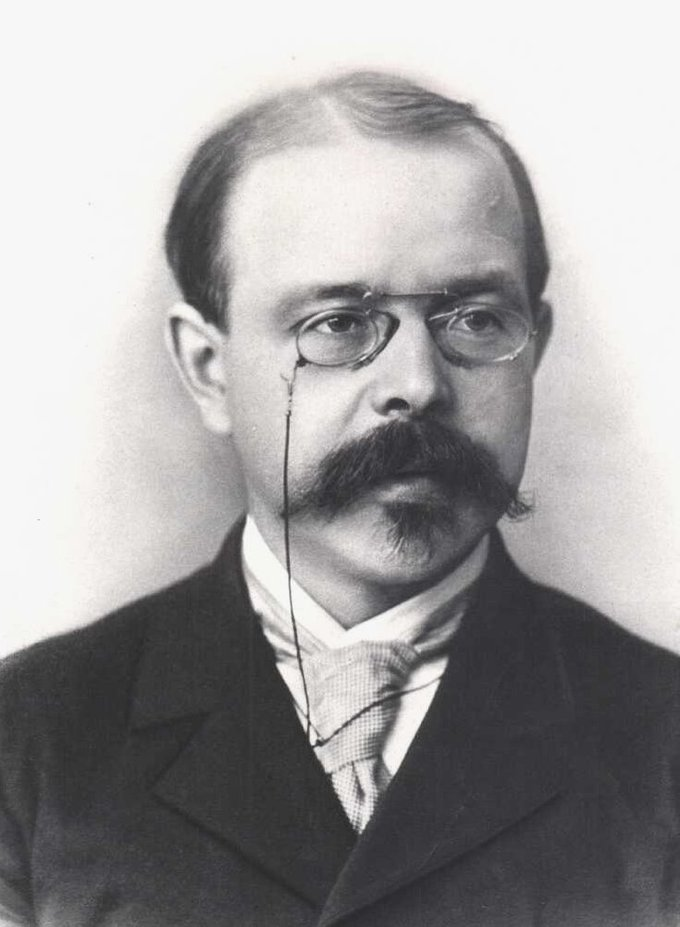## Thermodynamics of Redox Reactions

#### Learning Objective

• Translate between the equilibrium constant/reaction quotient, the standard reduction potential, and the Gibbs free energy change for a given redox reaction

#### Key Points

• Redox reactions are reactions that transfer electrons between species.
• The Nernst equation allows us to calculate the reduction potential of a redox reaction under “non-standard” conditions.
• When the system is at equilibrium, the Nernst equation can be simplified and written in terms of the equilibrium constant, K, which, in turn, allows for derivation of an equation to determine the change in Gibbs free energy ( $\Delta G$ ) of the reaction.

#### Terms

• standard reduction potentialThe reduction potential of a reaction measured under standard conditions: 25 °C, a 1 M concentration for each participating ion, a partial pressure of 1 atm for each gas, and metals in pure states.
• Gibbs free energyA thermodynamic potential that measures the “useful” or process-initiating work obtainable from a thermodynamic system at a constant temperature and pressure.

## Reduction Potential

In order to calculate thermodynamic quantities like change in Gibbs free energy $\Delta G$ for a general redox reaction, an equation called the Nernst equation must be used. Walther Nernst was a German chemist and physicist who developed an equation in the early 20th century to relate reduction potential, temperature, concentration, and moles of electrons transferred.Walther NernstA portrait of Walther Nernst.

The Nernst equation allows the reduction potential to be calculated at any temperature and concentration of reactants and products; the standard reaction potential must be measured at 298K and with each solution at 1M.

The Nernst equation is:

$E = E^0 - (\frac{RT}{nF})\ln Q$

Where the terms are as follows:

• E is the reduction potential for the specified non-standard state
• E0 is the standard reduction potential
• R and F are the gas and Faraday constants, respectively
• n is the number of electrons transferred in the reaction
• Q is the reaction quotient $\frac{C^cD^d}{A^aB^b}$. The uppercase letters are concentrations and the lowercase letters are stoichiometric coefficients for the reaction $aA + bB \rightarrow cC + dD$

If T is held constant at 298K, the Nernst equation can be condensed using the values for the constants R and F:

$E = E^0 - (\frac{0.0257}{n})\ln Q$

It can be further simplified if the reaction has reached equilibrium, as in that case Q is the equilibrium constant K:

$\ln K = \frac{nE^0}{0.0257}$

This equation allows the equilibrium constant to be calculated just from the standard reduction potential and the number of electrons transferred in the reaction.

## Gibbs Free Energy Charge

The relationship between the Gibbs free energy change and the standard reaction potential is:

$\Delta G = -nFE^0$

In this equation:

• $\Delta G$ is the change in free energy
• n is the number of moles
• E0 is the standard potential
• F is the Faraday constant